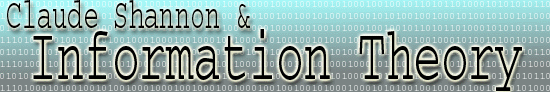Channel Capacity The channel capacity is the amount of information that can be processed per unit time over a noisy channel. In a channel, uncertainty is detrimental to information transfer, so the capacity is equal to 1 - H, where H is the entropy associated with a single bit. Information can be sent with a vanishing error rate while maintaining the information rate at the channel capacity. This is one of Shannon's most important results. However, he does not tell the exact encoding required to achieve this arbitrarily small error rate while maintaining the information rate at channel capacity. If the information rate from the source exceeds the channel capacity, then a message cannot be sent with an arbitrarily small error rate. This is a formula for the entropy associated with a digital channel. H = - ( p*log2p + q*log2q ) where p is the probability of a binary digit getting through unchanged and q is the probability of a binary digit being changed by the channel.  Note that p + q = 1. H is measured in bits per binary digit The question arises as to why channel capacity is 1 - H as opposed to H. The reason is that in the channel, we are looking for certainty as opposed to uncertainty, or entropy. Entropy in the source means that more information is being transmitted. However, the problem in the channel is to move the information from the source transmitter to the receiver intact. Therefore any uncertainty will result in less information being carried by a binary digit. You can think of each binary digit as lacking H bits of information in a channel with entropy H. This lost information must then be added back into the code in the form of check bits. If the fraction of the binary digits we sacrifice to error checking is at least H, then it is possible to correct the errors. Since C = 1 - H, we can encode a message in such a way that 100 * (1 - H) % of the binary digits encode the message, and 100 * H % of the binary digits are devoted to error checking. Theoretically, there exists such a code with a vanishing error rate. An intuitive justification for this is relatively easy to find. Consider a channel with a probability of error of 0.01. Also, take the following statement on faith: the laws of probability describe a system better and better as the sampling gets larger and larger. By taking larger and larger message lengths, it is more and more likely that the message that is received will deviate only 1% from the message that is sent. It can be shown that there are only 2^(n*H) messages that are likely to be received as the message length tends to Infiniti. This greatly reduces the amount of error-checking we must do. Although an error-rate of zero is impossible in this case, it can be made arbitrarily small by taking larger and larger message lengths. The fact that information can be transmitted essentially error-free at capacity is called the Noisy Channel Coding Theorem of Shannon. Before 1948, it was generally accepted that it was impossible to transmit error-free over a noisy channel with a non-zero information rate. Shannon proved that it was possible, but did not outline how. Achieving his limits in various situations has led to most ingenious solutions.